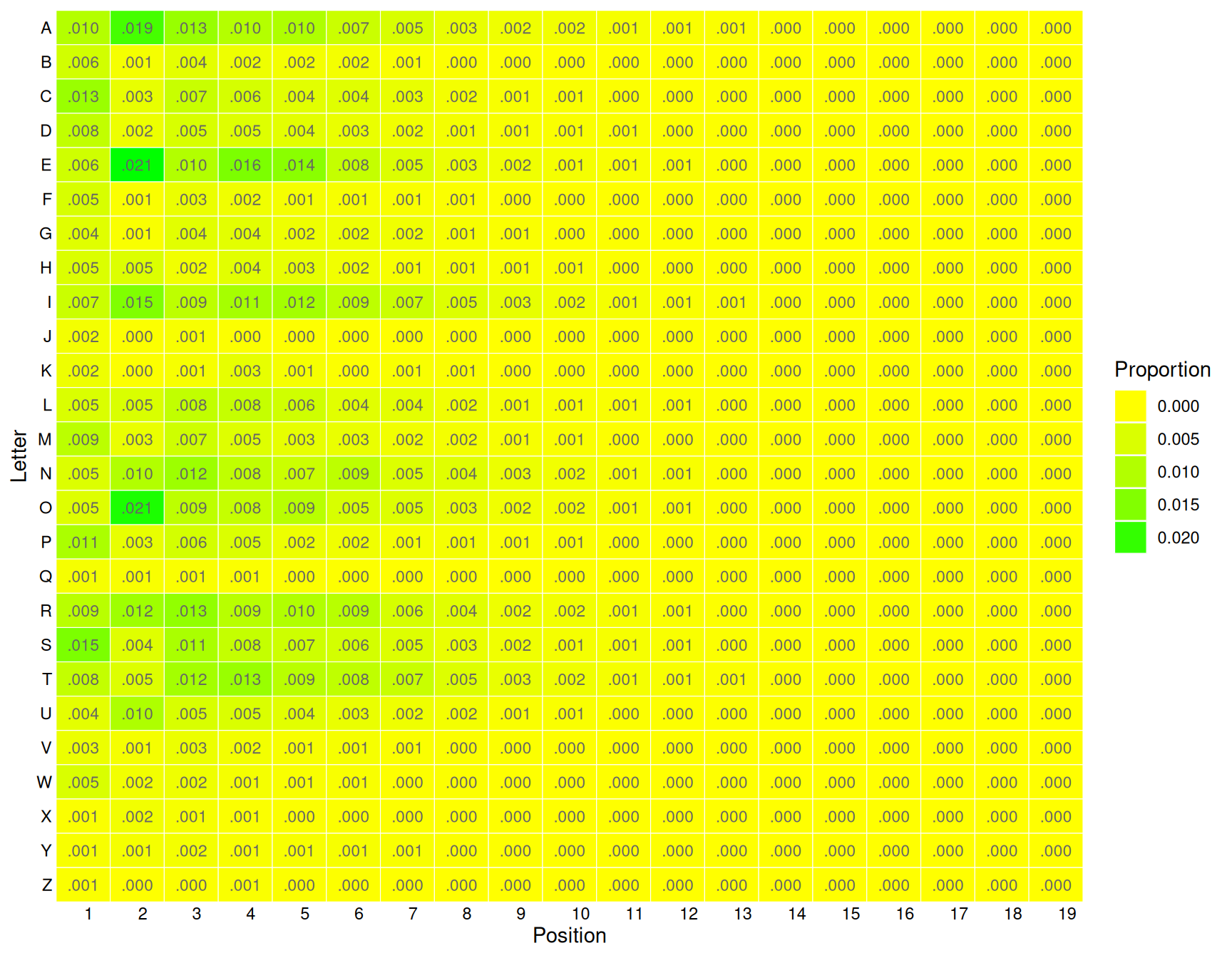## 21.36 Letter and Position Heatmap

## Warning: Ignoring unknown aesthetics: fillThe qdap::qheat() function from provides an effective visualisation of tabular data. Here we transform the list of words into a position count of each letter, and constructing a table of the proportions that is passed on to qdap::qheat() to do the plotting.

words                                                                 %>%
lapply(function(x) sapply(letters, gregexpr, x, fixed=TRUE))        %>%
unlist                                                              %>%
(function(x) x[x!=-1])                                              %>%
(function(x) setNames(x, gsub("\\d", "", names(x))))                %>%
(function(x) apply(table(data.frame(letter=toupper(names(x)),
position=unname(x))),
1, function(y) y/length(x)))                     %>%
qheat(high="green", low="yellow", by.column=NULL,
values=TRUE, digits=3, plot=FALSE)                             +
labs(y="Letter", x="Position") +
theme(axis.text.x=element_text(angle=0))                             +
guides(fill=guide_legend(title="Proportion"))

Your donation will support ongoing development and give you access to the PDF version of this book. Desktop Survival Guides include Data Science, GNU/Linux, and MLHub. Books available on Amazon include Data Mining with Rattle and Essentials of Data Science. Popular open source software includes rattle, wajig, and mlhub. Hosted by Togaware, a pioneer of free and open source software since 1984.